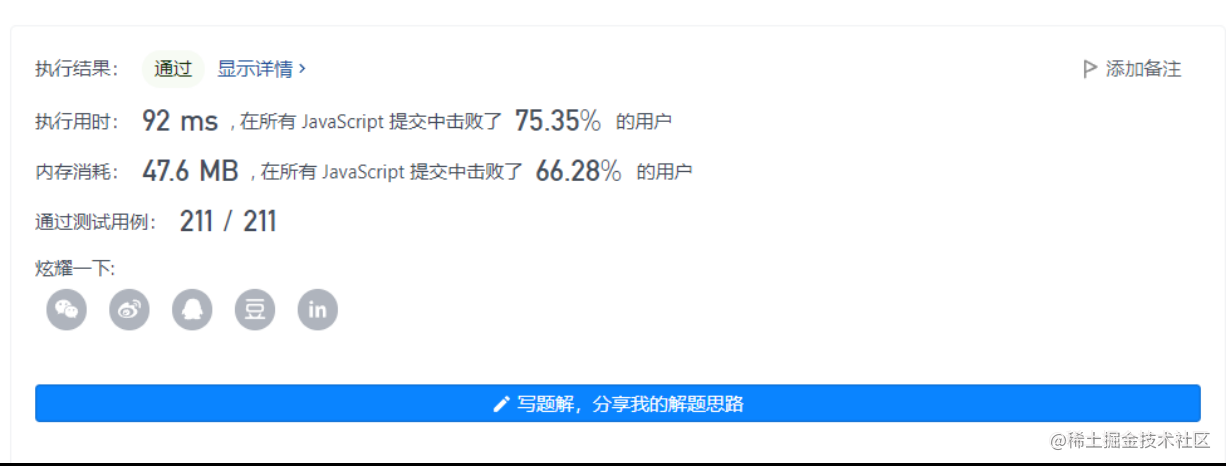2022-11-1700

## 目录

1. 题目
2. 解一：暴力法
3. 解二：动态规划(Dynamic programming)

# 1. 题目

``````输入：[7,1,5,3,6,4]

注意利润不能是 7-1 = 6, 因为卖出价格需要大于买入价格；同时，你不能在买入前卖出股票。
``````

``````输入：prices = [7,6,4,3,1]

``````

• `1 <= prices.length <= 105`
• `0 <= prices[i] <= 104`

# 2. 解一：暴力法

```javascript```/**
* @param {number[]} prices
* @return {number}
*/
var maxProfit = function(prices) {
let result = 0
const len = prices.length
for(let i = 0; i < len - 1; i++) {
for(let j = i + 1; j < len; j++) {
const earnings = prices[j] - prices[i]
if(earnings > result) {
result = earnings
}
}
}
return result
};
``````

• 时间复杂度：O(n2)
• 空间复杂度：O(1)可以看到直接超时了

# 3. 解二：动态规划(Dynamic programming)

```javascript```/**
* @param {number[]} prices
* @return {number}
*/
var maxProfit = function(prices) {
let inDay = maxNum = 0
let outDay = 1
const len = prices.length
for(let i = 1;i < len; i++,outDay++) {
const earnings = prices[outDay] - prices[inDay]
maxNum = Math.max(earnings, maxNum)

if(prices[i] < prices[inDay] ) {
inDay = outDay = i
}

}
return maxNum
};
``````

• 时间复杂度：O(n)
• 空间复杂度：O(1)